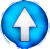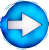First degree equation;: A term that changes member changes sign Fractions: Multiply the 2 members by the nominators least common multipleGo !

 $1 + x = 3$ $x = 3-1\qquad : \qquad S=\{2\}$ $3+x=-x+3$ $x+x = 3-3 \qquad;\qquad 2x = 0\qquad ;\qquad S =\{0\}$ $3+x=x+3$ $x-x = 3-3 \qquad;\qquad 0x = 0\qquad ;\qquad S = \mathbb R$ ( All real numbers are solution !)